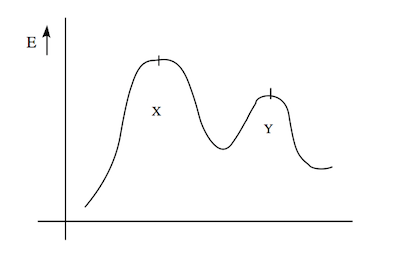Problem: For a 2 step reaction represented by the following reaction energy diagram which of the following statements is true? A)        Reaction is fast, product is in high yields B)        Reaction is fast, product is in low yields C)        The second step is fast and product is formed in low yields D)        The forward reaction has better yields than the reverse reaction

FREE Expert Solution
90% (133 ratings)
Problem Details

For a 2 step reaction represented by the following reaction energy diagram which of the following statements is true?

A)        Reaction is fast, product is in high yields

B)        Reaction is fast, product is in low yields

C)        The second step is fast and product is formed in low yields

D)        The forward reaction has better yields than the reverse reactionWhat scientific concept do you need to know in order to solve this problem?

Our tutors have indicated that to solve this problem you will need to apply the Energy Diagram concept. You can view video lessons to learn Energy Diagram Or if you need more Energy Diagram practice, you can also practice Energy Diagram practice problems .

What is the difficulty of this problem?

Our tutors rated the difficulty of For a 2 step reaction represented by the following reaction ... as medium difficulty.

How long does this problem take to solve?

Our expert Organic tutor, Chris took 7 minutes to solve this problem. You can follow their steps in the video explanation above.

What professor is this problem relevant for?

Based on our data, we think this problem is relevant for Professor Staff's class at MSU.